# Two monuments

The distance between two historical monuments on a map with a scale of 1: 500000 is 48 mm. Find the actual distance between these monuments in km.

x =  24 km

### Step-by-step explanation: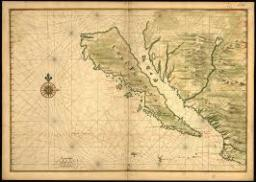Did you find an error or inaccuracy? Feel free to write us. Thank you!Tips to related online calculators
Do you want to convert length units?

## Related math problems and questions:

• Map scale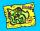What is the scale of the map, if 5.4 km is 72 mm on the map?
• Scale of the map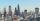The distance between two cities is actually 30 km and the map is 6 cm. What is the scale of the map?
• Scale of the mapDetermine the map's scale, which is the actual distance of 120 km l represented by a segment long 6 cm.
• Two villages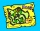On the map with a scale of 1:40000 are drawn two villages actually 16 km away. What is their distance on the map?
• Plan of the villagePlan of the municipality in 1:1000 scale has plotted garden with dimensions 25 mm and 28 mm. Determine the area of gardens in ares in reality.
• Geometric plan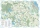At what scale the building plan if one side of the building is 45m long and 12mm long on a plan?
• Car model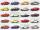Car model is 52 mm long and the real car is 4524 mm long. What was the scale of model car?
• The sidewalk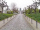The sidewalk is 50 m long. What will be its length on the plot plan on a scale of 1: 200?
• On the mapA line 1.5 cm long corresponds to a line 3 cm long on the map. What is the scale of the map?
• The plan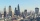The plan of the housing estate is in three scales 1: 5000,1: 10000,1: 15000. The distance between two points on a plan with a scale of 1: 10000 is 12 cm. What is this distance on the other two plans? What is this distance?
• Scale of the mapDetermine the scale of the map if the actual distance between A and B is 720 km and the distance on the map is 20 cm.
• Scale of planOn the plan of the village in the scale of 1: 1000 a rectangular garden is drawn. Its dimensions on the plan are 25mm and 28mm. Determine the area of the garden in ares.
• The scaleThe distance of 15 cm on the map corresponds to a distance of 27 km in reality. What is the scale of the map?
• Small city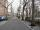The length of the street is 230 m. What will be the length of its image on the plan at a scale of 1: 2500?
• Two citiesOn a map, 1.5 cm represents 50 miles. If the distance between the two cities on the map is 6 cm, how many miles separate the cities?
• The distance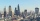The distance between two towns on a given map is 3 ½ cm. If ½ cm represents 6km. , what is the distance between the two towns?
• Map 2At what scale is made map if the distance 8.2 km corresponds on the map segment 5 cm long?